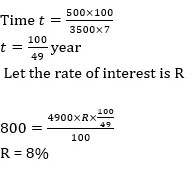# Quant Quiz for IBPS CLERK MAINS 2020 |18 January 2020

## Quant Quiz for IBPS CLERK MAINS:

Quant Quiz to improve your Quantitative Aptitude for SBI Po & SBI clerk exam IBPS PO Reasoning , IBPS Clerk Reasoning , IBPS RRB Reasoning, LIC AAO ,LIC Assistant  and other competitive exams

Q1. Paramesh invested an amount of Rs 12000 at the simple interest rate of 10 per cent annum and another amount at the simple interest rate of 20 per cent per annum. The total interest earned at the end of one year on the total amount invested became 14 per cent per annum. Find the total amount invested.

A) Rs 22000

B) Rs 25000

C) Rs 20000

D) Rs 24000

E) None of these

Q2. Anshul deposited two parts of a sum of Rs. 25000 in different banks at the rates of 13% per annum and 19% per annum respectively. In one year he got Rs. 3790 as the total interest. What was the amount deposited at the rate of 19% per annum?

A) Rs. 9000

B) Rs. 18000

C) Rs. 15000

D) Rs. 10000

E)  None of these

Q3. A person invests money in 3 different schemes for 3 years, 5 years and 6 years at 10%, 12% and 15% simple interest respectively. At the completion of each scheme, he gets the same interest. The ratio of his investments is

A) 2:3:6

B) 2:5:6

C) 6:5:2

D)  6:5:3

E) 6 : 3 : 2

Q4. Rs. 6100 was partly invested in Scheme A at 10% p.a. compound interest (compounded annually) for 2 years and partly in Scheme B at 10% p.a. simple interest for 4 years. Both the schemes give equal interests. How much was invested in Scheme A ?

A) Rs. 3750

B) Rs. 4500

C) Rs. 4000

D) Rs. 3250

E)  Rs. 5000

Q5. Amit puts some amount in bank and obtained an interest of Rs. 15 at the end of one year. He added Rs. 85 to his amount and put the whole amount again in the bank for another year. At the end of the 2nd year, Amit got Rs. 420 as total (interest + principal). What amount did he put in the beginning and what is the rate of interest offered by the bank if the minimum amount that can be deposited in the bank is Rs. 75 ?

A) 250, 5%

B)  300, 5%

C) 200, 8%

D) 350, 3.5%

E) None of these

Q6.  Rakesh borrows Rs. 3500 from a bank at SI. After three years he paid Rs. 1500 to the bank and at the end of 5 years from the date of borrowing he paid Rs. 2725 to the bank to settle the account. Find the rate of interest.

A) 10%

B) 5%

C) 2.5%

D) 3.5%

E) None of these

Q7. Ashok borrowed some money at simple interest at the rate of 6 per cent per annul for the first two years, at the rate of 9 per cent per annul for the next three years and at the rate of 14% per cent per annul for the period beyond five years. If he pays a total interest of Rs. 11400 at the end of 9 years how much money did he borrow ?

(a) Rs. 16,000

(b) Rs. 14,000

(c) Rs. 18,000

(d) Rs. 12,000

(e) None of these

Q8. On Rs 3500 invested at a simple interest rate 7 per cent per annum, Rs 500 is obtained as interest in certain years, In order to earn Rs 800 as interest on Rs 4900 in the same number of years, what should be the rate of simple interest?

(a) 9%

(b) 10%

(c) 12%

(d) 8%

(e) None of these

Q9. A person invested a certain amount at simple interest at the rate of 6 per cent per annul earning Rs. 900 as an interest at the end of three years. Had the interest been compounded every year, how much more interest would he have earned on the same amount with the same interest rate after three years ?

(a) Rs. 38.13

(b) Rs. 25.33

(c) Rs. 55.08

(d) Rs. 35.30

(e) None of these

Q10. If Rs.1000 be invested at interest rate of 5% and the interest be added to the principle every 10 years, then In how many years  it will amount to Rs. 2000 ?## Solutions

Q1. Ans(C)Q2. Ans(A)

Let the amount deposited at the rate of 19% per annum be x.Q3. Ans(E)Q4. Ans(C)Q5. Ans(B)Q6. Ans(B)Q7. Ans(d)Q8. Ans(d)Q9. Ans(c)Q10. Ans(a)### Online Mock Test Available on App as well as Web:### How to Access on App:-

1. Go to Playstore search Ambitious Baba or Click here to Install App

### You Can Read This Also:

ambitiousbaba.com need your support to Grow

I challenge you will get Best Content in Our PDFs with Detail solutions and Latest Pattern

Memory Based Puzzle E-book | 2016-19 Exams Covered

Get PDF here

Caselet Data Interpretation 200 Questions

Get PDF here
Puzzle & Seating Arrangement E-Book for BANK PO MAINS (Vol-1)

Get PDF here

### ARITHMETIC DATA INTERPRETATION 2019 E-book

Get PDF here
The Banking Awareness 500 MCQs E-book| Bilingual (Hindi + English)

Get PDF here

High Level DATA INTERPRETATION Practice E-BOOK

Get PDF her

3